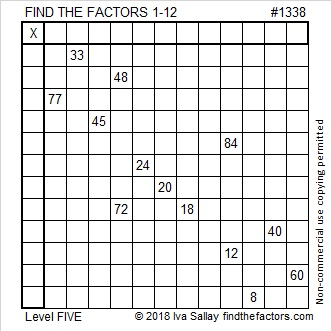# 1338 and Level 5

Finding the most logical place to start a level 5 puzzle is only a little bit trickier than for a level 4 puzzle. Will you figure it out and not be tricked?Print the puzzles or type the solution in this excel file: 12 factors 1333-1341

Here is some information about the number 1338:

• 1338 is a composite number.
• Prime factorization: 1338 = 2 × 3 × 223
• 1338 has no exponents greater than 1 in its prime factorization, so √1338 cannot be simplified.
• The exponents in the prime factorization are 1, 1, and 1. Adding one to each exponent and multiplying we get (1 + 1)(1 + 1)(1 + 1) = 2 × 2 × 2 = 8. Therefore 1338 has exactly 8 factors.
• The factors of 1338 are outlined with their factor pairs in the graphic below.1338 is in a couple of Pythagorean triples:
1338-447560-447562 calculated from 2(669)(1), 669² – 1², 669² + 1² and
1338-49720-49738 calculated from 2(223)(3), 223² – 3², 223² + 3²

This site uses Akismet to reduce spam. Learn how your comment data is processed.